#### 无线通信  >> Vol. 8 No. 4 (August 2018)

DOI: 10.12677/HJWC.2018.84023, PDF, HTML, XML, 下载: 617  浏览: 2,497

Abstract: The wireless ad hoc network has the advantages of flexible layout, non-essential for fixed equip-ment support and the information can be forwarded by other user nodes. It has been widely used in the harsh environment with emergency communication demand. This paper designs a MU-MIMO wireless ad-hoc network under the opportunistic relay scene. Then, the optimal pre-coding scheme is studied by using the semi-definite relaxation (SDR) algorithm. In addition, considering the high complexity of implementing the optimal solution, two sub-optimal pre-coding programs are also discussed, denoted as singular value decomposition and block diagonalization. Finally, the simulation results show that the optimal pre-coding scheme designed in this paper can improve the performance of the system.

1. 引言

2. 系统模型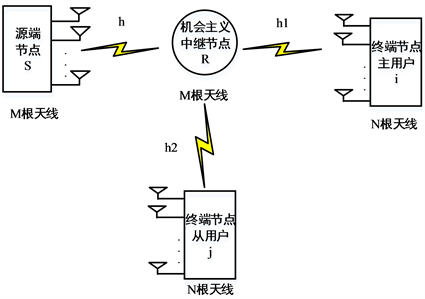Figure 1. MU-MIMO model of wireless self organizing network system

$y=hx+z$ (1)

$R={\mathrm{log}}_{2}|I+{\stackrel{¯}{\Lambda }}_{\rho }^{1/2}h{S}_{s}{h}^{H}{\stackrel{¯}{\Lambda }}_{\rho }^{1/2}|$ (2)

$P=tr\left({\Lambda }_{\rho }h{S}_{S}{h}^{H}\right)$ (3)

$\begin{array}{l}\text{P}1:\text{\hspace{0.17em}}\text{\hspace{0.17em}}\text{\hspace{0.17em}}\text{\hspace{0.17em}}\text{\hspace{0.17em}}\underset{\rho ,{S}_{S}}{\mathrm{max}}\text{}tr\left({\Lambda }_{\rho }h{S}_{s}{h}^{H}\right)\\ \text{\hspace{0.17em}}\text{\hspace{0.17em}}\text{\hspace{0.17em}}\text{\hspace{0.17em}}\text{\hspace{0.17em}}\text{\hspace{0.17em}}\text{\hspace{0.17em}}\text{\hspace{0.17em}}\text{\hspace{0.17em}}\text{\hspace{0.17em}}\text{s}\text{.t}\text{.}{r}_{R}={\mathrm{log}}_{2}|I+{\stackrel{¯}{\Lambda }}_{\rho }^{1/2}h{S}_{s}{h}^{H}{\stackrel{¯}{\Lambda }}_{\rho }^{1/2}|\text{}\ge \gamma \\ \text{\hspace{0.17em}}\text{\hspace{0.17em}}\text{\hspace{0.17em}}\text{\hspace{0.17em}}\text{\hspace{0.17em}}\text{\hspace{0.17em}}\text{\hspace{0.17em}}\text{\hspace{0.17em}}\text{\hspace{0.17em}}\text{\hspace{0.17em}}\text{\hspace{0.17em}}\text{\hspace{0.17em}}\text{\hspace{0.17em}}\text{\hspace{0.17em}}\text{ }\text{\hspace{0.17em}}tr\left({S}_{s}\right)\le {P}_{s}\\ \text{}\text{\hspace{0.17em}}\text{\hspace{0.17em}}\text{\hspace{0.17em}}\text{\hspace{0.17em}}\text{\hspace{0.17em}}\text{ }\text{\hspace{0.17em}}{S}_{s}\ge 0\\ \text{\hspace{0.17em}}\text{\hspace{0.17em}}\text{\hspace{0.17em}}\text{\hspace{0.17em}}\text{\hspace{0.17em}}\text{\hspace{0.17em}}\text{\hspace{0.17em}}\text{\hspace{0.17em}}\text{\hspace{0.17em}}\text{\hspace{0.17em}}\text{\hspace{0.17em}}\text{\hspace{0.17em}}\text{\hspace{0.17em}}\text{\hspace{0.17em}}\text{ }\text{\hspace{0.17em}}0\le \rho \le 1\end{array}$ (4)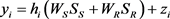(5)

${y}_{j}={h}_{j}\left({W}_{S}{S}_{S}+{W}_{R}{S}_{R}\right)+{z}_{j}$ (6)

$SIN{R}_{i}=\frac{{|{h}_{i}{W}_{s}|}^{2}}{{|{h}_{i}{W}_{R}|}^{2}+{\sigma }_{i}^{2}}$ (7)

$SIN{R}_{j}=\frac{{|{h}_{j}{W}_{R}|}^{2}}{{|{h}_{j}{W}_{s}|}^{2}+{\sigma }_{j}^{2}}$ (8)

$\begin{array}{l}\text{P}2:\text{\hspace{0.17em}}\text{\hspace{0.17em}}\text{\hspace{0.17em}}\text{\hspace{0.17em}}\underset{{W}_{R},{W}_{S}}{\mathrm{max}}\text{\hspace{0.17em}}\text{\hspace{0.17em}}\text{\hspace{0.17em}}\frac{{|{h}_{j}{W}_{R}|}^{2}}{{|{h}_{j}{W}_{s}|}^{2}+{\sigma }_{j}^{2}}\\ \text{\hspace{0.17em}}\text{\hspace{0.17em}}\text{\hspace{0.17em}}\text{\hspace{0.17em}}\text{\hspace{0.17em}}\text{\hspace{0.17em}}\text{\hspace{0.17em}}\text{\hspace{0.17em}}\text{\hspace{0.17em}}\text{s}\text{.t}\text{.}\text{\hspace{0.17em}}\text{\hspace{0.17em}}\text{\hspace{0.17em}}\text{\hspace{0.17em}}\text{\hspace{0.17em}}\frac{{|{h}_{i}{W}_{s}|}^{2}}{{|{h}_{i}{W}_{R}|}^{2}+{\sigma }_{i}^{2}}\ge \psi \\ \text{\hspace{0.17em}}\text{\hspace{0.17em}}\text{\hspace{0.17em}}\text{\hspace{0.17em}}\text{\hspace{0.17em}}\text{\hspace{0.17em}}\text{\hspace{0.17em}}\text{\hspace{0.17em}}\text{\hspace{0.17em}}\text{\hspace{0.17em}}\text{\hspace{0.17em}}\text{\hspace{0.17em}}\text{\hspace{0.17em}}\text{\hspace{0.17em}}\text{\hspace{0.17em}}\text{\hspace{0.17em}}\text{\hspace{0.17em}}{‖{W}_{S}‖}^{2}+{‖{W}_{R}‖}^{2}\le P\end{array}$ (9)

3. 最优化解法

$\begin{array}{l}\underset{{S}_{S}}{\mathrm{max}}\text{}tr\left({\Lambda }_{\stackrel{¯}{\rho }}h{S}_{s}{h}^{H}\right)\\ \text{s}\text{.t}\text{.}{r}_{R}={\mathrm{log}}_{2}|I+{\stackrel{¯}{\Lambda }}_{\stackrel{¯}{\rho }}^{1/2}h{S}_{s}{h}^{H}{\stackrel{¯}{\Lambda }}_{\stackrel{¯}{\rho }}^{1/2}|\ge \gamma \\ \text{\hspace{0.17em}}\text{\hspace{0.17em}}\text{\hspace{0.17em}}\text{\hspace{0.17em}}\text{\hspace{0.17em}}\text{ }tr\left({S}_{s}\right)\le {P}_{s}\\ \text{}\text{\hspace{0.17em}}{S}_{s}\ge 0\end{array}$ (10)

$\begin{array}{l}\text{P2-SDR}:\text{\hspace{0.17em}}\underset{S,Q}{\mathrm{max}}\text{\hspace{0.17em}}\text{\hspace{0.17em}}\text{\hspace{0.17em}}\frac{Tr\left({H}_{j}Q\right)}{Tr\left({H}_{j}S\right)+{\sigma }_{j}^{2}}\\ \text{\hspace{0.17em}}\text{\hspace{0.17em}}\text{\hspace{0.17em}}\text{\hspace{0.17em}}\text{\hspace{0.17em}}\text{\hspace{0.17em}}\text{\hspace{0.17em}}\text{\hspace{0.17em}}\text{\hspace{0.17em}}\text{\hspace{0.17em}}\text{\hspace{0.17em}}\text{\hspace{0.17em}}\text{\hspace{0.17em}}\text{\hspace{0.17em}}\text{s}\text{.t}\text{.}\text{\hspace{0.17em}}\text{\hspace{0.17em}}\text{\hspace{0.17em}}\text{\hspace{0.17em}}Tr\left({H}_{i}S\right)\ge \psi \left(Tr\left({H}_{i}Q\right)+{\sigma }_{i}^{2}\right),\\ \text{\hspace{0.17em}}\text{\hspace{0.17em}}\text{\hspace{0.17em}}\text{\hspace{0.17em}}\text{\hspace{0.17em}}\text{\hspace{0.17em}}\text{\hspace{0.17em}}\text{\hspace{0.17em}}\text{\hspace{0.17em}}\text{\hspace{0.17em}}\text{\hspace{0.17em}}\text{\hspace{0.17em}}\text{\hspace{0.17em}}\text{\hspace{0.17em}}\text{\hspace{0.17em}}\text{\hspace{0.17em}}\text{\hspace{0.17em}}\text{\hspace{0.17em}}\text{\hspace{0.17em}}\text{\hspace{0.17em}}\text{\hspace{0.17em}}\text{ }\text{ }Tr\left(S\right)+Tr\left(Q\right)\le P,\\ \text{\hspace{0.17em}}\text{\hspace{0.17em}}\text{\hspace{0.17em}}\text{\hspace{0.17em}}\text{\hspace{0.17em}}\text{\hspace{0.17em}}\text{\hspace{0.17em}}\text{\hspace{0.17em}}\text{\hspace{0.17em}}\text{\hspace{0.17em}}\text{\hspace{0.17em}}\text{\hspace{0.17em}}\text{\hspace{0.17em}}\text{\hspace{0.17em}}\text{\hspace{0.17em}}\text{\hspace{0.17em}}\text{\hspace{0.17em}}\text{\hspace{0.17em}}\text{\hspace{0.17em}}\text{\hspace{0.17em}}\text{\hspace{0.17em}}\text{\hspace{0.17em}}S\ge 0,\text{\hspace{0.17em}}\text{\hspace{0.17em}}Q\ge 0\end{array}$ (11)

$\begin{array}{l}\text{P2-SDR-Equ}:\text{\hspace{0.17em}}\underset{S,Q,t}{\mathrm{max}}\text{\hspace{0.17em}}\text{\hspace{0.17em}}\text{\hspace{0.17em}}Tr\left({H}_{j}Q\right)\\ \text{\hspace{0.17em}}\text{\hspace{0.17em}}\text{\hspace{0.17em}}\text{\hspace{0.17em}}\text{\hspace{0.17em}}\text{\hspace{0.17em}}\text{\hspace{0.17em}}\text{\hspace{0.17em}}\text{\hspace{0.17em}}\text{\hspace{0.17em}}\text{\hspace{0.17em}}\text{\hspace{0.17em}}\text{\hspace{0.17em}}\text{\hspace{0.17em}}\text{\hspace{0.17em}}\text{\hspace{0.17em}}\text{\hspace{0.17em}}\text{\hspace{0.17em}}\text{\hspace{0.17em}}\text{\hspace{0.17em}}\text{s}\text{.t}\text{.}\text{\hspace{0.17em}}\text{\hspace{0.17em}}\text{\hspace{0.17em}}\text{\hspace{0.17em}}Tr\left({H}_{j}S\right)+t{\sigma }_{j}^{2}=1,\\ \text{\hspace{0.17em}}\text{\hspace{0.17em}}\text{\hspace{0.17em}}\text{\hspace{0.17em}}\text{\hspace{0.17em}}\text{\hspace{0.17em}}\text{\hspace{0.17em}}\text{\hspace{0.17em}}\text{\hspace{0.17em}}\text{\hspace{0.17em}}\text{\hspace{0.17em}}\text{\hspace{0.17em}}\text{\hspace{0.17em}}\text{\hspace{0.17em}}\text{\hspace{0.17em}}\text{\hspace{0.17em}}\text{\hspace{0.17em}}\text{\hspace{0.17em}}\text{\hspace{0.17em}}\text{\hspace{0.17em}}\text{\hspace{0.17em}}\text{}\text{\hspace{0.17em}}\text{ }Tr\left({H}_{i}S\right)\ge \psi \left(Tr\left({H}_{i}Q\right)+t{\sigma }_{i}^{2}\right),\\ \text{\hspace{0.17em}}\text{\hspace{0.17em}}\text{\hspace{0.17em}}\text{\hspace{0.17em}}\text{\hspace{0.17em}}\text{\hspace{0.17em}}\text{\hspace{0.17em}}\text{\hspace{0.17em}}\text{\hspace{0.17em}}\text{\hspace{0.17em}}\text{\hspace{0.17em}}\text{\hspace{0.17em}}\text{\hspace{0.17em}}\text{\hspace{0.17em}}\text{\hspace{0.17em}}\text{\hspace{0.17em}}\text{\hspace{0.17em}}\text{\hspace{0.17em}}\text{\hspace{0.17em}}\text{\hspace{0.17em}}\text{\hspace{0.17em}}\text{}\text{ }\text{\hspace{0.17em}}Tr\left(S\right)+Tr\left(Q\right)\le tP,\\ \text{\hspace{0.17em}}\text{\hspace{0.17em}}\text{\hspace{0.17em}}\text{\hspace{0.17em}}\text{\hspace{0.17em}}\text{\hspace{0.17em}}\text{\hspace{0.17em}}\text{\hspace{0.17em}}\text{\hspace{0.17em}}\text{\hspace{0.17em}}\text{\hspace{0.17em}}\text{\hspace{0.17em}}\text{\hspace{0.17em}}\text{\hspace{0.17em}}\text{\hspace{0.17em}}\text{\hspace{0.17em}}\text{\hspace{0.17em}}\text{\hspace{0.17em}}\text{\hspace{0.17em}}\text{\hspace{0.17em}}\text{\hspace{0.17em}}\text{}\text{\hspace{0.17em}}\text{ }S\ge 0,\text{\hspace{0.17em}}\text{\hspace{0.17em}}Q\ge 0,\text{\hspace{0.17em}}t>0\end{array}$ (12)

1) P2-SDR的所有可行解为(S,Q)，其对应的问题P2-SDR-Equ解为

$\left(\frac{S}{Tr\left({H}_{j}S\right)+{\sigma }_{j}^{2}},\frac{Q}{Tr\left({H}_{j}S\right)+{\sigma }_{j}^{2}},\frac{1}{Tr\left({H}_{j}S\right)+{\sigma }_{j}^{2}}\right)$ ，可以看出两个问题有同样的目标值S与Q。

2) 问题P2-SDR-Equ对应的可行解为(S,Q,t)，而P2-SDR中的可行解为(S/t,Q/t)，两个问题的目标值相同，因此两个问题是等价的。

4. 次优化解法

4.1. 通过奇异值分解(SVD)设计第一时隙中次优化预编码矩阵

${y}_{R}=\sqrt{{P}_{s}}hFx+n$ (13)

$r=G\left(\sqrt{{P}_{s}}hFx+n\right)=Dx+{U}^{H}n$ (14)

$P=\zeta \left(1-\rho \right){P}_{s}{|hF|}^{2}$ (15)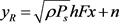(16)

$SNR=\frac{\rho {P}_{s}{|hF|}^{2}}{{\sigma }_{R}^{2}}$ (17)

$\begin{array}{l}\text{P1-1}:\underset{\rho }{\mathrm{max}}\text{\hspace{0.17em}}\text{\hspace{0.17em}}\text{\hspace{0.17em}}\text{\hspace{0.17em}}\text{\hspace{0.17em}}\zeta \left(1-\rho \right){P}_{s}{|hF|}^{2}\text{\hspace{0.17em}}\text{\hspace{0.17em}}\text{\hspace{0.17em}}\text{\hspace{0.17em}}\text{\hspace{0.17em}}\text{ }\text{\hspace{0.17em}}\text{\hspace{0.17em}}\text{\hspace{0.17em}}\text{ }\text{ }\left(18\text{-}1\right)\\ \text{\hspace{0.17em}}\text{\hspace{0.17em}}\text{\hspace{0.17em}}\text{\hspace{0.17em}}\text{\hspace{0.17em}}\text{\hspace{0.17em}}\text{\hspace{0.17em}}\text{\hspace{0.17em}}\text{\hspace{0.17em}}\text{s}\text{.t}\text{\hspace{0.17em}}\text{\hspace{0.17em}}\text{\hspace{0.17em}}\text{\hspace{0.17em}}\frac{\rho Ps{|hF|}^{2}}{{\sigma }_{R}^{2}}\ge \psi \text{\hspace{0.17em}}\text{\hspace{0.17em}}\text{\hspace{0.17em}}\text{\hspace{0.17em}}\text{\hspace{0.17em}}\text{\hspace{0.17em}}\text{\hspace{0.17em}}\text{\hspace{0.17em}}\text{\hspace{0.17em}}\text{\hspace{0.17em}}\text{\hspace{0.17em}}\text{ }\text{\hspace{0.17em}}\text{\hspace{0.17em}}\text{\hspace{0.17em}}\text{\hspace{0.17em}}\left(18\text{-}2\right)\\ \text{\hspace{0.17em}}\text{\hspace{0.17em}}\text{\hspace{0.17em}}\text{\hspace{0.17em}}\text{\hspace{0.17em}}\text{\hspace{0.17em}}\text{\hspace{0.17em}}\text{\hspace{0.17em}}\text{\hspace{0.17em}}\text{\hspace{0.17em}}\text{\hspace{0.17em}}\text{\hspace{0.17em}}\text{\hspace{0.17em}}\text{\hspace{0.17em}}\text{\hspace{0.17em}}\text{\hspace{0.17em}}\text{\hspace{0.17em}}\zeta \left(1-\rho \right){P}_{s}{|hF|}^{2}\le Ps\text{\hspace{0.17em}}\text{\hspace{0.17em}}\text{\hspace{0.17em}}\text{}\left(18\text{-}3\right)\\ \text{\hspace{0.17em}}\text{\hspace{0.17em}}\text{\hspace{0.17em}}\text{\hspace{0.17em}}\text{\hspace{0.17em}}\text{\hspace{0.17em}}\text{\hspace{0.17em}}\text{\hspace{0.17em}}\text{\hspace{0.17em}}\text{\hspace{0.17em}}\text{\hspace{0.17em}}\text{\hspace{0.17em}}\text{\hspace{0.17em}}\text{\hspace{0.17em}}\text{\hspace{0.17em}}\text{\hspace{0.17em}}\text{\hspace{0.17em}}0<\rho <1\text{\hspace{0.17em}}\text{\hspace{0.17em}}\text{\hspace{0.17em}}\text{\hspace{0.17em}}\text{\hspace{0.17em}}\text{\hspace{0.17em}}\text{\hspace{0.17em}}\text{\hspace{0.17em}}\text{\hspace{0.17em}}\text{\hspace{0.17em}}\text{\hspace{0.17em}}\text{\hspace{0.17em}}\text{\hspace{0.17em}}\text{\hspace{0.17em}}\text{\hspace{0.17em}}\text{\hspace{0.17em}}\text{\hspace{0.17em}}\text{\hspace{0.17em}}\text{\hspace{0.17em}}\text{\hspace{0.17em}}\text{\hspace{0.17em}}\text{\hspace{0.17em}}\text{\hspace{0.17em}}\left(18\text{-}4\right)\end{array}$ (18)

${\rho }^{*}\text{\hspace{0.17em}}\text{and}\text{\hspace{0.17em}}{\lambda }^{*},\text{\hspace{0.17em}}{\nu }^{*}$ 分别是(18-2)和(18-3)的拉格朗日乘子，问题P1-1的KKT条件为：

${{\nabla }_{\rho }L\left(\rho ,\lambda ,\nu \right)|}_{{\rho }^{*},{\lambda }^{*},{\nu }^{*}}=0$ (19)

$\left\{\begin{array}{l}{\lambda }^{*}\left(\frac{\rho Ps{|hF|}^{2}}{{\sigma }_{R}^{2}}-\psi \right)=0\\ {\nu }^{*}\left(\varsigma \left(1-\rho \right)Ps{|hF|}^{2}-Ps\right)=0\end{array}$ (20)

 (21)

$\left\{\begin{array}{l}\frac{{\rho }^{*}Ps{|hF|}^{2}}{{\sigma }_{R}^{2}}-\psi \ge 0\\ \varsigma \left(1-{\rho }^{*}\right)Ps{|hF|}^{2}-Ps\le 0\end{array}$ (22)

P1-1的拉格朗日表达式 $L\left(•\right)$ 为：

$\begin{array}{c}L\left(\rho ,\lambda ,\nu \right)=\varsigma \left(1-\rho \right)Ps{|hF|}^{2}-\lambda \left(\frac{\rho Ps{|hF|}^{2}}{{\sigma }_{R}^{2}}-\psi \right)\\ \text{\hspace{0.17em}}\text{\hspace{0.17em}}+\nu \left(\varsigma \left(1-\rho \right)Ps{|hF|}^{2}-Ps\right)\end{array}$ (23)

${{\nabla }_{\rho }L\left(\rho ,\lambda ,\nu \right)|}_{{\rho }^{*},{\lambda }^{*},{\nu }^{*}}=-\varsigma Ps{|hF|}^{2}+{\lambda }^{*}\frac{Ps{|hF|}^{2}}{{\sigma }_{R}^{2}}=0$ (24)

4.2. 通过块对角化(BD)设计第二时隙中次优化预编码矩阵

${h}_{j}={U}_{j}{\Lambda }_{j}{\left[{V}_{j}^{\left(1\right)}{V}_{j}^{\left(0\right)}\right]}^{H}$ (25)

${h}_{i}{V}_{j}^{\left(0\right)}={U}_{i}^{\left(b\right)}{\Lambda }_{i}^{\left(b\right)}{V}_{i}^{\left(b\right)}{}^{H}$ (26)

${h}_{i}={U}_{i}{\Lambda }_{i}{\left[{V}_{i}^{\left(1\right)}{V}_{i}^{\left(0\right)}\right]}^{H}$ (27)

${h}_{j}{V}_{i}^{\left(0\right)}={U}_{j}^{\left(b\right)}{\Lambda }_{j}^{\left(b\right)}{V}_{j}^{\left(b\right)}{}^{H}$ (28)

$tr\left({W}_{S}\right)+tr\left({W}_{R}\right)\le P$ (29)

$\begin{array}{c}{y}_{i}={M}_{i}{h}_{i}\left({W}_{S}{S}_{i}+{W}_{R}{S}_{j}\right)+{n}_{i}\\ ={M}_{i}{h}_{i}\left({V}_{j}^{\left(0\right)}{V}_{i}^{\left(b\right)}{\Phi }_{i}^{\frac{1}{2}}{S}_{i}+{V}_{i}^{\left(0\right)}{V}_{j}^{\left(b\right)}{\Phi }_{j}^{\frac{1}{2}}{S}_{j}\right)+{n}_{i}\\ ={\Lambda }_{1}^{\left(b\right)}{\Phi }_{i}^{\frac{1}{2}}{S}_{i}+{n}_{i}\end{array}$ (30)

$SIN{R}_{i}=\frac{{\left({\Lambda }_{i}^{\left(b\right)}\right)}^{2}{\Phi }_{i}}{{\sigma }_{i}^{2}}$ (31)

$\begin{array}{c}{y}_{j}={M}_{j}{h}_{j}\left({W}_{S}{S}_{i}+{W}_{R}{S}_{j}\right)+{n}_{j}\\ ={M}_{j}{h}_{j}\left({V}_{j}^{\left(0\right)}{V}_{i}^{\left(b\right)}{\Phi }_{i}^{\frac{1}{2}}{S}_{i}+{V}_{i}^{\left(0\right)}{V}_{j}^{\left(b\right)}{\Phi }_{j}^{\frac{1}{2}}{S}_{j}\right)+{n}_{j}\\ ={\Lambda }_{j}^{\left(b\right)}{\Phi }_{j}^{\frac{1}{2}}{S}_{j}+{n}_{j}\end{array}$ (32)

$SIN{R}_{j}=\frac{{\left({\Lambda }_{2}^{\left(b\right)}\right)}^{2}{\Phi }_{j}}{{\sigma }_{j}^{2}}$ (33)

$\begin{array}{l}\text{P2-SubOp}:\text{\hspace{0.17em}}\text{\hspace{0.17em}}\text{\hspace{0.17em}}\text{\hspace{0.17em}}\underset{{\Phi }_{i},{\Phi }_{j}}{\mathrm{max}}\text{\hspace{0.17em}}\text{\hspace{0.17em}}\text{\hspace{0.17em}}\text{\hspace{0.17em}}\text{\hspace{0.17em}}\frac{{\left({\Lambda }_{j}^{\left(b\right)}\right)}^{2}{\Phi }_{j}}{{\sigma }_{j}^{2}}\\ \text{\hspace{0.17em}}\text{\hspace{0.17em}}\text{\hspace{0.17em}}\text{\hspace{0.17em}}\text{\hspace{0.17em}}\text{\hspace{0.17em}}\text{\hspace{0.17em}}\text{\hspace{0.17em}}\text{\hspace{0.17em}}\text{\hspace{0.17em}}\text{\hspace{0.17em}}\text{\hspace{0.17em}}\text{\hspace{0.17em}}\text{\hspace{0.17em}}\text{\hspace{0.17em}}\text{\hspace{0.17em}}\text{\hspace{0.17em}}\text{\hspace{0.17em}}\text{\hspace{0.17em}}\text{\hspace{0.17em}}\text{s}\text{.t}.\text{\hspace{0.17em}}\text{\hspace{0.17em}}\text{\hspace{0.17em}}\text{\hspace{0.17em}}\text{\hspace{0.17em}}\frac{{\left({\Lambda }_{i}^{\left(b\right)}\right)}^{2}{\Phi }_{i}}{{\sigma }_{i}^{2}}\ge \gamma \\ \text{\hspace{0.17em}}\text{\hspace{0.17em}}\text{\hspace{0.17em}}\text{\hspace{0.17em}}\text{\hspace{0.17em}}\text{\hspace{0.17em}}\text{\hspace{0.17em}}\text{\hspace{0.17em}}\text{\hspace{0.17em}}\text{\hspace{0.17em}}\text{\hspace{0.17em}}\text{\hspace{0.17em}}\text{\hspace{0.17em}}\text{\hspace{0.17em}}\text{\hspace{0.17em}}\text{\hspace{0.17em}}\text{\hspace{0.17em}}\text{\hspace{0.17em}}\text{\hspace{0.17em}}\text{\hspace{0.17em}}\text{\hspace{0.17em}}\text{\hspace{0.17em}}\text{\hspace{0.17em}}\text{\hspace{0.17em}}\text{\hspace{0.17em}}\text{\hspace{0.17em}}\text{ }\text{\hspace{0.17em}}\text{\hspace{0.17em}}tr\left({W}_{S}\right)+tr\left({W}_{R}\right)\le P\\ \text{\hspace{0.17em}}\text{\hspace{0.17em}}\text{\hspace{0.17em}}\text{\hspace{0.17em}}\text{\hspace{0.17em}}\text{\hspace{0.17em}}\text{\hspace{0.17em}}\text{\hspace{0.17em}}\text{\hspace{0.17em}}\text{\hspace{0.17em}}\text{\hspace{0.17em}}\text{\hspace{0.17em}}\text{\hspace{0.17em}}\text{\hspace{0.17em}}\text{\hspace{0.17em}}\text{\hspace{0.17em}}\text{\hspace{0.17em}}\text{\hspace{0.17em}}\text{\hspace{0.17em}}\text{\hspace{0.17em}}\text{\hspace{0.17em}}\text{\hspace{0.17em}}\text{\hspace{0.17em}}\text{\hspace{0.17em}}\text{\hspace{0.17em}}\text{\hspace{0.17em}}\text{\hspace{0.17em}}\text{\hspace{0.17em}}{\Phi }_{i}>0,\text{\hspace{0.17em}}{\Phi }_{j}>0\end{array}$ (34)

5. 仿真分析

1) 主用户最低速率需求越大，从用户的总速率越小。这是因为中继节点在第一时隙中接收到的能量有限，主用户对速率的需求越高，中继节点可以分配给从用户的功率就越小，因此影响从用户的传输速率。

2) 通过波束赋形算法设计的最优化预编码矩阵在给定的两种情况下都表现出了最优性能。原因是波束赋形能够将发射信号的主瓣对应接收信号的到达角度，旁瓣对应干扰信号的到达角度，在能够增强有用信号的同时还能在一定程度上消除干扰信号，从而提高系统速率。但是最优化方案的实现复杂度很高，因此次优化解目前更具现实意义。

3) 在用户端的天线数量小于发射端天线数量时，块对角化预编码的效果与最优化方案非常接近，这是由于块对角化算法预编码可以将干扰信号映射到有效信号的零空间，从而达到干扰消除效果。块对角化算法预编码在用户数量较少，信道状态已知的条件下很容易实现预编码矩阵设计，计算复杂度较低。而采用奇异值分解算法进行预编码设计，会导致用户空间的子信道成90度，分解后子信道性能差异较大，因此效果相对较差。

1) 当用户端天线数量等于发射端时，块对角化算法的性能最差，这是因为随着天线数量的增多，信道维度变大，此时块对角化算法不能很好的消除用户间信道干扰，因此性能较差。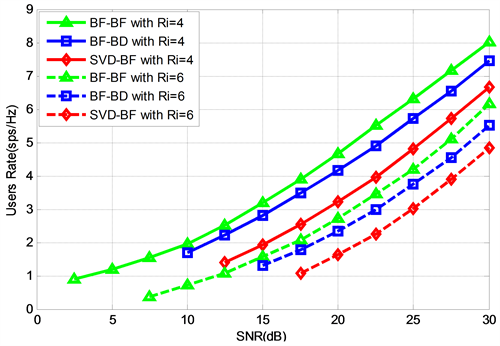Figure 2. The system rate of the number of antennas at N = 4, M = 2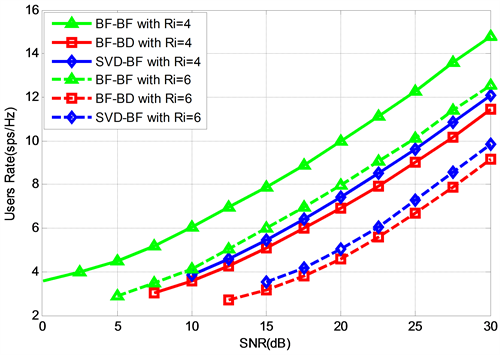Figure 3. The system rate of the number of antennas at N = 4, M = 4

2) 用户端天线数量的增加显著地增强了系统的容量，最优化方案效果更佳明显。这是因为波束赋形算法不受分离的子信道性能及接收端天线数目的影响，且MIMO系统的吞吐量随天线数目增多而线性增长，因此波束赋形算法速率提升最大。

6. 结语

  Sudevalayam, S. and Kulkarni, P. (2011) Energy Harvesting Sensor Nodes: Survey and Implications. IEEE Communications Surveys & Tutorials, 13, 443-461. https://doi.org/10.1109/SURV.2011.060710.00094  Chen, H., Xiao, L., Yang, D., Zhang, T. and Cuthbert, L. (2017) User Cooperation in Wireless Powered Communication Networks with a Pricing Mechanism. IEEE Access, 5, 16895-16903. https://doi.org/10.1109/ACCESS.2017.2740403  Yang, D.C., Zhu, C.Q., Xiao, L., Shen, X.M. and Zhang, T.K. (2016) An Energy-Efficient Scheme for Multirelay Cooperative Networks with Energy Harvesting. Mobile Information Systems, No. 5618935.  Sadek, A.K., Liu, K.J.R. and Ephremides, A. (2007) Cognitive Multiple Access via Cooperation: Protocol Design and Stability Analysis. IEEE Transactions on Information Theory, 53, 3677-3696. https://doi.org/10.1109/TIT.2007.904784  Grover, P. and Sahai, A. (2010) Shannon Meets Tesla: Wireless Information and Power Transfer. IEEE International Symposium on Information Theory (ISIT), Austin, 13-18 June 2010, 2363-2367. https://doi.org/10.1109/ISIT.2010.5513714  Yang, D.C., Zhou, X.X., Xiao, L. and Wu, F.H. (2015) Energy Cooperation in Multi-Users Wireless-Powered Relay Networks. IET Communications, 9, 1412-1420.  Chen, H., Xiao, L., Yang, D., Zhang, T. and Cuthbert, L. (2017) User Cooperation in Wireless Powered Communication Networks with a Pricing Mechanism. IEEE Access, 5, 16895-16903. https://doi.org/10.1109/ACCESS.2017.2740403  Liu, L., Zhang, R. and Chua, K.C. (2013) Wireless Information and Power Transfer: A Dynamic Power Splitting Approach. IEEE Transactions on Communications, 61, 3990-4001. https://doi.org/10.1109/TCOMM.2013.071813.130105  Khandaker, M.R.A. and Wong, K.K. (2014) QoS-Based Multicast Beamforming for SWIPT. 2014 Eleventh Annual IEEE International Conference on Sensing, Communication, and Networking Workshops (SECON Workshops), Singapore, Singapore, 30 June-3 July 2014, 62-67. https://doi.org/10.1109/SECONW.2014.6979707  Devroye, N., Mitran, P. and Tarokh, V. (2006) Achievable Rates in Cognitive Radio Channels. IEEE Transactions on Information Theory, 52, 1813-1827. https://doi.org/10.1109/TIT.2006.872971  Yang, D.C. (2015) Wireless Information and Power Transfer: Optimal Power Control in One-Way and Two-Way Relay System. Wireless Personal Communications, 84, 1-14. https://doi.org/10.1007/s11277-015-2874-4  Yang, D.C., Zhou, X.X. and Xiao, L. (2015) Energy Cooperation in Multi-User Wireless-Powered Relay Networks. IET Communications, 9, 1412-1420. https://doi.org/10.1049/iet-com.2014.1100  Bi, S., Ho, C.K. and Zhang, R. (2015) Wireless Powered Communication: Opportunities and Challenges. IEEE Communications Magazine, 53, 117-125. https://doi.org/10.1109/MCOM.2015.7081084  Grant, M. and Boyd, S. (2011) CVX: Matlab Software for Disciplined Convex Programming, Version 1.21. http://cvxr.com/cvx/